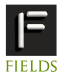11-15 July 2011 INTERNATIONAL CONFERENCE ON SCIENTIFIC COMPUTATION AND DIFFERENTIAL EQUATIONS SciCADE 2011 hosted by the Fields Institute, held at the University of TorontoProgram New Talent Award Toronto Information Sign up for E-lerts programs(PUT_AT_SIGN_HERE)fields.utoronto.ca Contact Us gensci(PUT_AT_SIGN_HERE)fields.utoronto.caMINISYMPOSIA Numerical differential equations and their applications, II organized by Anita Layton, Duke University Wayne Hayes (Imperial College London) Ned Nedialkov (McMaster University)
This minisymposium, to be held in honor of Professor Kenneth Jackson's 60th birthday, will survey novel discretization techniques in numerical differential equations that address the computational challenges posed by high index, low regularity, long-time integration, etc. Topics include mathematical finance, simulations of the solar system, computational fluid dynamics, and medical imaging.

 Meng Han, University of Toronto A novel approximation to loss probabilities of credit portfolios Credit risk analysis and management at the portfolio level are challenging problems for financial institutions due to their portfolios' large size, heterogeneity and complex correlation structure. The conditional-independence framework is widely used to calculate loss probabilities of credit portfolios. The existing computational approaches within this framework fall into two categories: (1) simulation-based approximations and (2) asymptotic approximations. The simulation-based approximations often involve a two-level Monte Carlo method, which is extremely time-consuming, while the asymptotic approximations, which are typically based on the Law of Large Number (LLN), are not accurate enough for tail probabilities, especially for heterogeneous portfolios. We give a more accurate asymptotic approximation based on the Central Limit Theorem (CLT), and we discuss when it can be applied. To further increase accuracy, we also propose a hybrid approximation, which combines the simulation-based approximation and the asymptotic approximation. We test our approximations with some artificial and real portfolios. Numerical examples show that, for a similar computational cost, the CLT approximation is more accurate than the LLN approximation for both homogeneous and heterogeneous portfolios, while the hybrid approximation is even more accurate than the CLT approximation. Moreover, the hybrid approximation significantly reduces the computing time for comparable accuracy compared to simulation-based approximations. Nargol Rezvani University of Toronto A polyenergetic model and iterative reconstruction framework for X-ray computerized tomography (CT) While most modern x-ray CT scanners rely on the well-known filtered back-projection (FBP) algorithm, the corresponding reconstructions can be corrupted by beam-hardening artifacts. These artifacts arise from the unrealistic physical assumption of monoenergetic x-ray beams. To compensate, we discretize an alternative model directly that accounts for differential absorption of polyenergetic x-ray photons. We present numerical reconstructions based on the associated nonlinear discrete formulation incorporating various iterative optimization frameworks. This is joint work with D. Aruliah and K. Jackson. Dongwoon Lee University of Toronto Geometric reconstruction and physical simulation of human skeletal muscle Data from human cadavers enable us to obtain in-depth understanding and accurate parameter estimation of human skeletal muscle. However, as most muscles have non-homogeneous architecture, it is not straightforward to determine their parameters. Thus, we propose a geometric approach in which volumes of fiber bundles are individually approximated as parametric solids and aggregated to determine the architecture and geometry of human skeletal muscle. Lumped-parameter models are commonly used in many computational studies of human skeletal muscle. However, the simplified representation of architectural parameters inherent in these models often limits their ability to determine morphology and mechanical functions of complex muscles. Therefore, we are developing micro-mechanical muscle models to incorporate variable architectural parameters and non-uniform fiber arrangements, enabling us to predict contractile behaviors of complex muscles. Duy Minh Dang University of Toronto An efficient GPU-based parallel algorithm for pricing multi-asset American options We develop highly-efficient parallel Partial Differential Equation (PDE) based pricing methods on Graphics Processing Units (GPUs) for multi-asset American options. Our pricing approach is built upon a combination of a discrete penalty approach for the linear complementarity problem arising due to the free boundary and a GPU-based parallel Alternating Direction Implicit Approximate Factorization technique with finite differences on uniform grids for the solution of the linear algebraic system arising from each penalty iteration. A timestep size selector implemented efficiently on GPUs is used to further increase the efficiency of the methods. We demonstrate the efficiency and accuracy of the parallel numerical methods by pricing American options written on three assets.